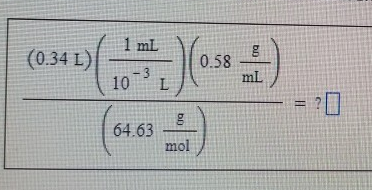# Problem: A student sets up the following equation to solve a problem in solution stoichiometry. Enter the units of the students answer.

###### FREE Expert Solution

We simply need to cancel units that appears both in the numerator and denominator:

90% (232 ratings)###### Problem Details

A student sets up the following equation to solve a problem in solution stoichiometry.

Enter the units of the students answer.Frequently Asked Questions

What scientific concept do you need to know in order to solve this problem?

Our tutors have indicated that to solve this problem you will need to apply the Dimensional Analysis concept. You can view video lessons to learn Dimensional Analysis. Or if you need more Dimensional Analysis practice, you can also practice Dimensional Analysis practice problems.